## Saturday, April 14, 2012

### How Does It Work, Part 1

Analytic geometry is one of the most useful kinds of mathematics I have ever learned. Painter was built on it. But the average person has little idea what makes it tick, much less what makes it relevant. This post will simplify two-dimensional analytic geometry and present it so anybody can understand it. I'll build you up from understanding points on the plane to segments and vectors. And then to angles, directions, orientation, area, really you name it (if it has to do with analytic geometry) and I can help you understand it.The Plane, the Plane!

Pixels and points are two ways of looking at the plane. But both of them involve a two-dimensional address system, and the two dimensions are usually called X and Y, but sometimes also columns and rows.

We see here a right-handed coordinate system. On the compass, the X-axis points east and the Y-axis points north. In the center is the origin, the point where X and Y are both zero. We like to write a coordinate as an ordered pair, in this case (0, 0). The axes also split the plane into four quadrants (shown in color and with their conventional Roman numerals).

If you are working in columns and rows instead of X and Y, then you are actually using a left-handed coordinate system, because larger rows are below the smaller ones, and so the direction of increasing rows points south. Pixels in an image are usually laid out in rows and columns.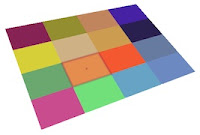A coordinate system is the basis for all analytic geometry. A point is an infinitely small location in the plane. A pixel is really a small rectangle covering a (usually square) area in the plane. The center of the pixel is a point.

Vegments and Sectors

Wait, I meant segments and vectors (I'm just trying to confuse you with doubletalk, hee hee)! Join two points with a small straight line and you get a segment. If you join two points in the same way, but the join actually has a direction, then you have a vector. Segments and vectors may be measured and quantified in different ways. And each has their own use.Here I show a segment from point P1 (X1, Y1) to P2 (X2, Y2). A segment is rooted (meaning it has a real position on the plane) because both endpoints have locations.

Segments may be chained end-to-end to create polygons. Most commonly, a polygon is closed, in which case it has an inside and an outside. You can compute a closed polygon's area also. More (much more!) on this later.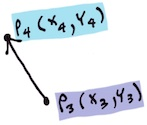Here is a vector from point P3 (X3, Y3) to point P4 (X4, Y4). But a vector, which we have conveniently defined to be the straight path between these two endpoints is really not rooted and consists of only an X and a Y delta. A vector is pretty much the same no matter where you put it.

A vector's measure starts by computing the X and Y deltas that make it up. Once you perform the subtraction, any notion of being rooted in the plane goes away. Why? This is intuitively true because you subtract away the start point of the vector, removing its home base.

Length and Stuff (No, It Isn't Spelled Lenght! Grrr!)

Simple subtraction creates the vector, as you can see. This is why a vector is oriented. A vector from P3 to P4 is quite different from the vector from P4 to P3. In fact, it is the negative of it. Or inverse.Its length may be easily calculated using Pythagoras' rule by making the segment the hypotenuse of a right triangle (you probably wondered when that rule was going to be useful to you in everyday life, right? Well, just wait until you get to trigonometry!).

So here is the easy way to compute the length of a segment. You move the "right angle" of the imaginary right triangle (kind of shown shaded in a very light peach color) directly to the origin. This translation is effectively performing a subtraction, in this case, of the lesser of the two X's, which happens to be X4, from the two points, and also a subtraction of the lesser of the two Y's, Y3, from the two points. Anyway, the base and height of the right triangle are now known, so we may compute its hypotenuse length using Pythagoras' rule.Note that, when you square them, the deltas that make up a vector can be either positive or negative. This is because their signs get lost when you square them: they always end up non-negative (also coincidentally why the square root of a negative number is not a real number). So we can define the length of the vector to be the square root of the sum of the squares of the vector's deltas.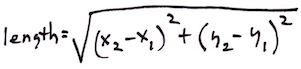If you imagine a segment to be a vector, then you can compute its length in exactly the same way. Length doesn't depend upon the position of the segment. That's why it works to think of the segment as a vector in this case.

OMG Do I Have To Know This Stuff?!?

If you already know about all the kinds of numbers, then you can skip this section. However, just bear in mind that I had to know about all these things before I could develop Painter! And now it's your turn! Waahahahahaa!

There are various kinds of numbers that it is useful to know. The integers are the whole numbers, zero, and their negatives. On a computer, there may be a smallest integer and a largest integer. That is what we like to call an implementation detail in the business. If you need more accuracy, you might want to use a real number. Real numbers can represent the integers, and all the numbers in between as well, like 9.57212947621.... On computers, these are represented by things called floats and doubles. These have various numbers of bits of accuracy.

When you divide one integer by another integer, you get a rational number (but please don't divide by zero!). There is really quite a spectrum of numbers that can be represented by rational numbers, called a Markov spectrum (but I digress). On a computer, real numbers can't really represent all rational numbers with 100% accuracy. For instance, you can represent 123/128 exactly, but 1/3 is hopeless to represent accurately. This turns out to be true because computers use power-of-two (binary) representations, and 128 just happens to be two to the seventh power.

Some numbers can't be represented exactly as a rational number or by floats and doubles on the computer. One example of these is an algebraic number: a number that is the root of some polynomial with integer coefficients, like the square root of two. If a segment has its endpoints at points whose X and Y are integers, then the segment's length is an algebraic number.

Another example is a transcendental number. Such a number can't be represented as an algebraic number or a rational number. Well, pi and e, the base of natural logarithms, are two examples of transcendental numbers.

OK, computers really only approximate these, but it's good enough for government work.

Back to the Vector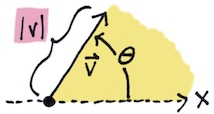A vector then has a length and also an angle. Mathematicians have for ages thought of angles as being rooted in a vector that points east. This is the zero angle, and it corresponds with the X axis. Angles increase counter-clockwise, starting at zero and increasing until they become 360 degrees back at the X axis again. Although mathematicians actually think in radians. A radian is about 57.3 degrees. This means that 360 degrees is actually two pi radians.

Pi, by the way, is the magic ratio of the circumference of a circle to its diameter. It has a value of about 3.14159.... Yes, I memorized pi to 100 places and I used to recite it. Jeez, was I a geek!

But radians are a pure mathematical way of looking at things. Using radians instead of degrees saves you a multiply each time you do a trig function. Uh-oh. Trig functions!Well, anyway, when you have a vector, and you divide its X and Y components by its length, you get a unit vector that points in the same direction. This unit vector consists of the cosine and the sine of the direction angle, by the way.

I remember when I was introduced to this fact. It was, to me, an "aha" moment. Now I understood why trigonometry was important! Actually, a few things fell into place that day in 1974. I ended up using it later on a test given to me by Joe Sukonick, a clever programmer at Calma Company. That bearded MIT graduate fellow ended up with a patent on the XOR for cursors in raster systems, by the way. But it was another guy at Calma, Tom Schaefer, that taught me some really useful things about analytic geometry. Without this information, it would have been seriously difficult to create Painter, or build the mosaic brush. Or really anything I do today.

What's Dot?

At Calma, segments definitely got connected end-to-end to create polygons. Calma built very good CAD (computer-aided design) systems for the very first VLSI (very large scale integration) components. In other words, microchips like the Motorola 68000 were designed on our systems. Yep, the original Macintosh's CPU.

I remember thinking about the notion of concave and convex and clockwise and counterclockwise. How could I determine these attributes for a polygon? It seemed like concavity was a local thing, and could be determined by looking at three consecutive points. But clockwise and counterclockwise were much more complicated, and were clearly a global thing. Or were they?

So there I was at the tender age of 19 at Calma, thinking abstractly about analytic geometry. It turns out I was smart enough and up to the challenge!Tom Schaefer clicked his pen a few times and then drew a few figures on a piece of Calma stationery. Tom Schaefer was responsible for the all-angle fracturing software that enabled e-beam devices to render arbitrary geometries onto silicon, so I believed he could help me.

First there were three points, P1, P2, and P3. You could subtract the points' X's and Y's to make two vectors, V1 and V2.

But note that, the two vectors, if rooted at the same origin, created an angle between them, called theta. The angle is measured in counterclockwise degrees (or radians!).That's when he introduced two operators, dot and codot. Dot is really the dot product and codot is sometimes called the scalar cross product. I found the operators' definition to be unusually simple and low-cost computationally. You just multiplied the X and Y components of the two vectors together in different ways. Wow!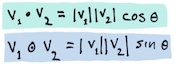Then he proceeded to show me that these operators could actually be used to compute the sine and the cosine of the angle theta. It was all beginning to come together. But I was a little hazy on my trig, so he made it absolutely clear to me. It's all about which side P2 is with respect to the line that passes through P0 and P1.In fact, the sign of the codot could be used to determine this useful bit of information. And here's how to do just that.

OMG I thought this was incredibly clever. With this one operation, I could decide if a polygon is concave or convex, and even whether to not a polygon was clockwise or counterclockwise (as long is it's not self-intersecting).

But, of course, my brain was thinking several steps ahead.

It also occurred to me that, if V1 and V2 were unit vectors, that the dot and codot operators would simply produce the cosine and the sine of theta directly. In fact, I could see now that the rule about a vector's angle was simply a special case of this.

The dot product, it turned out, was useful in determining whether the two vectors were pointing in the same basic direction (if the dot is positive) or the opposite direction (if the dot is negative). And, by the way, if the dot product is zero, then the two vectors are at right angles to each other (perpendicular).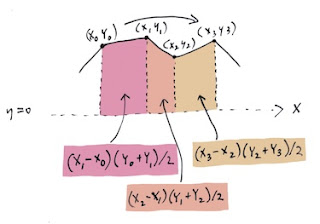Area, Orientation, and Lobster Bisque

Polygons are a connected and closed chain of points in the plane. Between any two neighboring points is a segment. So, how do we compute the area of a polygon? Well, we make use of a clever technique called the trapezoidal rule. If a polygon is clockwise, then we just have to make trapezoids from each segment by dropping lines to the Y axis as we see in this diagram. Note that, on the bottom of the polygon, when the segments are going in the other direction, then the trapezoid areas become negative, because the X terms are going from right-to-left instead of from left-to-right as they are here. And that makes polygon area work by first computing the integral under the top of the polygon and subtracting the integral below the bottom of the polygon.

This has an interesting side effect. The clockwise polygons have a positive area and the counterclockwise polygons have a negative area.

So, really, to get the area, we have to take the absolute value of the computed area. And the sign is the orientation of the polygon. This turns out to be a very convenient way to compute polygon area! Once you see it, you will wonder how it can be so simple.

This turned out to be a question on Joe Sukonick's aptitude test at Calma. I took that test, and I got it right. But another guy, Bruce Holloway, got the job. Because, I was going to college at Caltech. The very next spring, when in Chemistry lab at Caltech, I got a call from Art Collmeyer. He saw the test and decided I might be interested in a summer job at Calma. It's good he called. I met Tom Hedges at Calma.

Oh, and by the way, the lobster bisque at Hula's Grille in Santa Cruz is excellent!